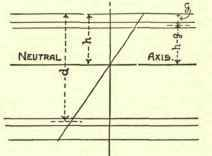In Fig. 59 the compressional reinforcement is shown at depth g below the surface, and according to the theory of similar triangles the strain at this point will h - g be h x strain at surface; consequently the stress in the reinforcement becomes r.c h - g = where r= Es h Ec

Let ac = sectional area of compressional reinforcement, and at = sectional area of tensile reinforcement.

Total compressional stress - total tensile stress -2 bhc + acc.r.h - g = atfr. 3 h a bh1c + 3 accr(h -g) ft= 3athAgain, h d - h= 1 ft/cr h= d

=1: 2bh1+ 3accr (h - g) 3athr1+ 2bh1 + 3ar(h-g).

3athrMoment of resistance = moment of resistance of concrete + moment of resistance of compressional reinforcement.Mr=2/3bhc (d-2/5h)+accr h-g/h (d-g).

A compressional reinforcement is particularly useful where a tee beam passes over a support; for here, where the stresses are reversed, the comparatively thin rib has to meet the compressional stress without the aid of the horizontal member which resisted compression at the centre of the span. This reinforcement is readily provided by simply carrying the members, which served as tensile reinforcement at the centre, straight along the lower side and over the supports. Further compressive resistance is provided at this point by the splaying out of the pillars immediately below the beam, as seen in Fig. 34. In using the formulæ just given it should not be forgotten that the use of compressional reinforcement adds to the strength of the concrete by bridging over weak points, as pointed out elsewhere; thus the latter formulae, as compared with those given on page 39, probably err on the side of safety.

## Shear

The necessity for the use of members to resist shear has already been discussed.

If the total vertical shear at any point in a beam's length = S, where depth of beam = d feet, the average horizontal shear at this point = S/d, while the maximum shear at neutral axis =3/2 x S/d, Vertical or in clined stirrups should be used, offering sufficient sectional area to resist this stress. The resistance of steel in shear may be taken as 11,000 lbs. per square inch.Fig. 59.

All stirrups used in a beam will, for convenience, be of equal dimensions, being placed next together at the supports where the shear is greatest, and farther apart at the centre of the span. In the Hennebique system the tensile reinforcements which are sloped up over the supports are usually considered as meeting half the shear at the ends, while stirrups are arranged to resist the remaining half.

Armoured or Reinforced Concrete for Various Uses 41